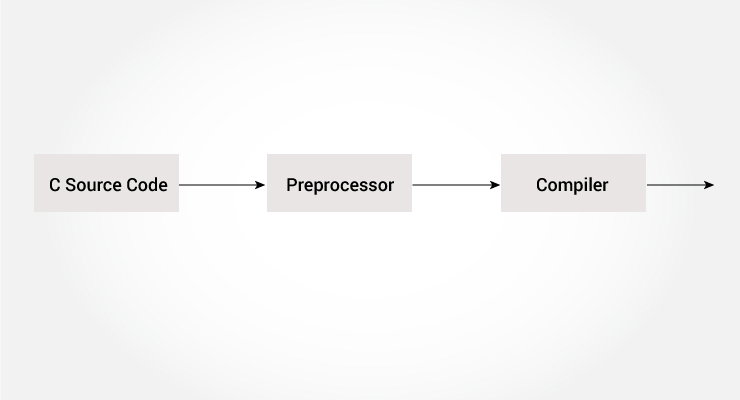C Preprocessor and Macros

# C Preprocessor and Macros

#### In this tutorial, you will be introduced to c preprocessors, and you will learn to use #include, #define and conditional compilation with the help of examples.The C preprocessor is a macro preprocessor (allows you to define macros) that transforms your program before it is compiled. These transformations can be the inclusion of header file, macro expansions etc.

All preprocessing directives begin with a `#` symbol. For example,

``#define PI 3.14``

Some of the common uses of C preprocessor are:

The `#include` preprocessor is used to include header files to C programs. For example,

``#include <stdio.h>``

Here, `stdio.h` is a header file. The `#include` preprocessor directive replaces the above line with the contents of `stdio.h` header file.

That's the reason why you need to use `#include <stdio.h>` before you can use functions like `scanf()` and `printf()`.

You can also create your own header file containing function declaration and include it in your program using this preprocessor directive.

``#include "my_header.h"``

## Macros using #define

A macro is a fragment of code that is given a name. You can define a macro in C using the `#define` preprocessor directive.

Here's an example.

``#define c 299792458  // speed of light``

Here, when we use c in our program, it is replaced with `299792458`.

### Example 1: #define preprocessor

``````#include <stdio.h>
#define PI 3.1415

int main()
{

// Notice, the use of PI

printf("Area=%.2f",area);
return 0;
}``````

## Function like Macros

You can also define macros that work in a similar way like a function call. This is known as function-like macros. For example,

``#define circleArea(r) (3.1415*(r)*(r))``

Every time the program encounters `circleArea(argument)`, it is replaced by `(3.1415*(argument)*(argument))`.

Suppose, we passed 5 as an argument then, it expands as below:

``circleArea(5) expands to (3.1415*5*5)``

### Example 2: Using #define preprocessor

``````#include <stdio.h>
#define PI 3.1415
#define circleArea(r) (PI*r*r)

int main() {

printf("Area = %.2f", area);

return 0;
}``````

## Conditional Compilation

In C programming, you can instruct preprocessor whether to include a block of code or not. To do so, conditional directives can be used.

It's similar to a `if` statement with one major difference.

The `if` statement is tested during the execution time to check whether a block of code should be executed or not whereas, the conditionals are used to include (or skip) a block of code in your program before execution.

### Uses of Conditional

• use different code depending on the machine, operating system
• compile same source file in two different programs
• to exclude certain code from the program but to keep it as reference for future purpose

### How to use conditional?

To use conditional, `#ifdef`, `#if`, `#defined`, `#else` and `#elseif` directives are used.

### #ifdef Directive

``````#ifdef MACRO
// conditional codes
#endif```
```

Here, the conditional codes are included in the program only if MACRO is defined.

### #if, #elif and #else Directive

``````#if expression
// conditional codes
#endif```
```

Here, expression is an expression of integer type (can be integers, characters, arithmetic expression, macros and so on).

The conditional codes are included in the program only if the expression is evaluated to a non-zero value.

The optional `#else` directive can be used with `#if` directive.

``````#if expression
conditional codes if expression is non-zero
#else
conditional if expression is 0
#endif```
```

You can also add nested conditional to your `#if...#else` using `#elif`

``````#if expression
// conditional codes if expression is non-zero
#elif expression1
// conditional codes if expression is non-zero
#elif expression2
// conditional codes if expression is non-zero
#else
// conditional if all expressions are 0
#endif```
```

### #defined

The special operator #defined is used to test whether a certain macro is defined or not. It's often used with #if directive.

``````#if defined BUFFER_SIZE && BUFFER_SIZE >= 2048
// codes```
```

## Predefined Macros

Here are some predefined macros in C programming.

Macro Value
`__DATE__` A string containing the current date
`__FILE__` A string containing the file name
`__LINE__` An integer representing the current line number
`__STDC__` If follows ANSI standard C, then the value is a nonzero integer
`__TIME__` A string containing the current date.

### Example 3: Get current time using __TIME__

The following program outputs the current time using `__TIME__` macro.

``````#include <stdio.h>
int main()
{
printf("Current time: %s",__TIME__);
}``````

Output

`Current time: 19:54:39`